Courses

# Analysis of Trusses Notes | EduRev

## GATE : Analysis of Trusses Notes | EduRev

The document Analysis of Trusses Notes | EduRev is a part of the GATE Course Structural Analysis.
All you need of GATE at this link: GATE

Trusses are used commonly in Steel buildings and bridges.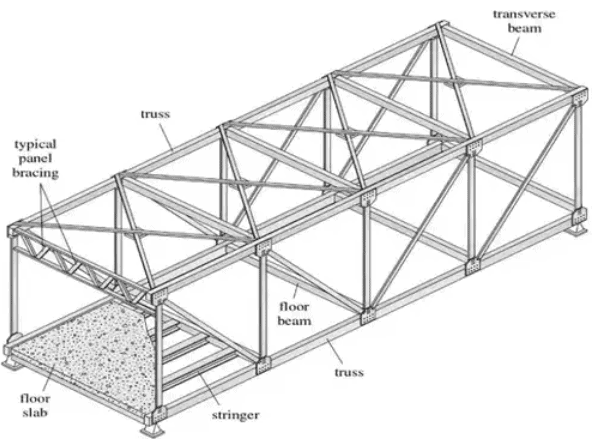Definition: A truss is a structure that consists of

• All straight members
• Connected together with pin joints
• Connected only at the ends of the members
• All external forces (loads & reactions) must be applied only at the joints.
• Trusses are assumed to be of negligible weight (compared to the loads they carry)

Types of Trusses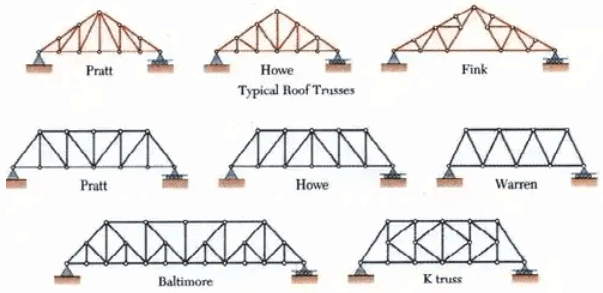Typical Bridge Trusses

Degree of Static Indeterminacy
• DS = m+re – 2j where, DS = Degree of static indeterminacy m = Number of members, re = Total external reactions, j = Total number of joints
• DS = 0 ⇒ Truss is determinate
• If Dse = + 1 & Dsi = –1 then DS = 0 at specified point.
• DS > 0 ⇒ Truss is indeterminate or dedundant.
Truss Analysis: Method of Joints
• Conditions of equilibrium are satisfied for the forces at each joint
• Equilibrium of concurrent forces at each joint
• Only two independent equilibrium equations are involved

Steps of Analysis

1. Draw Free Body Diagram of Truss
2. Determine external reactions by applying equilibrium equations to the whole truss
3. Perform the force analysis of the remainder of the truss by Method of Joints

Example 1: Determine the force in each member of the loaded truss by Method of Joints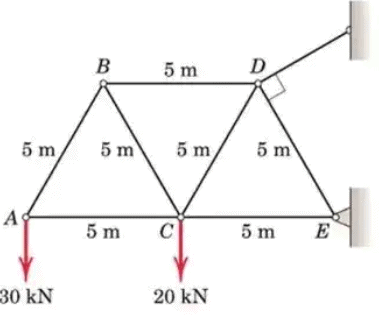Solution: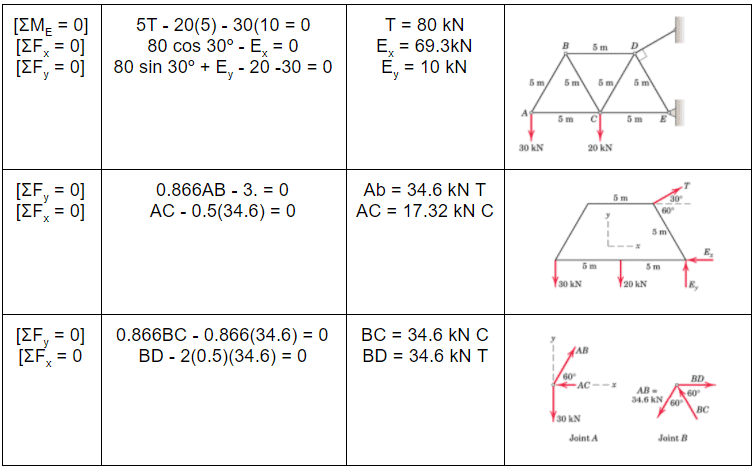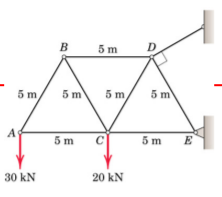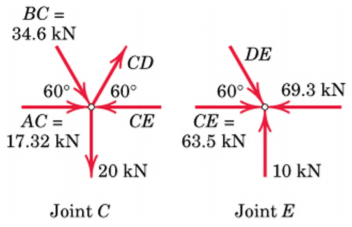[ΣFy = 0] 0.866BC - 0.866(34.6) - 20 = 0
Cd = 57.7 kn T
[ΣFx = 0 CE - 17.32 - 0.5(34.6) - 0.5(57.7) = 0
CE = 63.5 kN C
[ΣFy = 0] 0.866DE = 10 DE = 11.55 kN C
and the equation ΣF= 0 checks.

Truss Member Carrying Zero forces

(i) M1, M2, M3 meet at a joint M1 & M2 are collinear ⇒ Mcarries zero force where M1, M2, M3 represents member.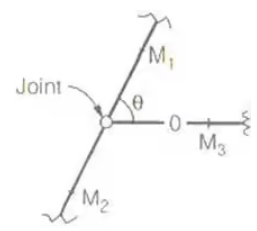(ii) M1 & M2 are non collinear and Fext = 0 ⇒ M1 & M2 carries zero force.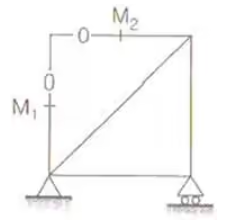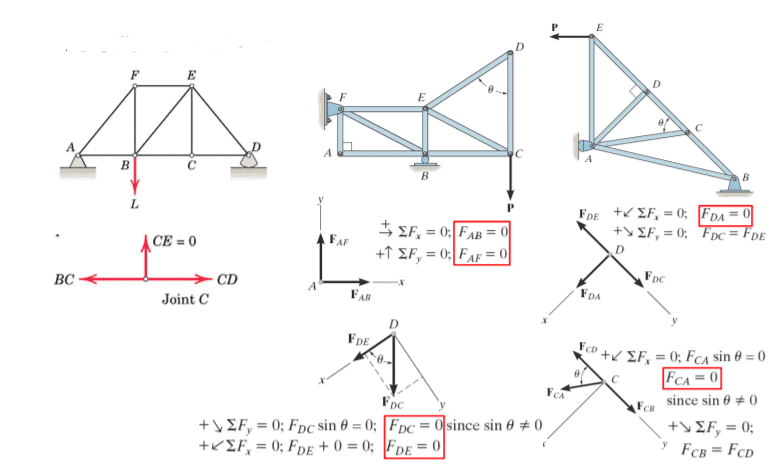• If only two non-collinear members form a truss joint and no external load or support reaction is applied to the joint, the two members must be zero force members
• If three members form a truss joint for which two of the members are collinear, the third member is a zero-force member provided no external force or support reaction is applied to the joint.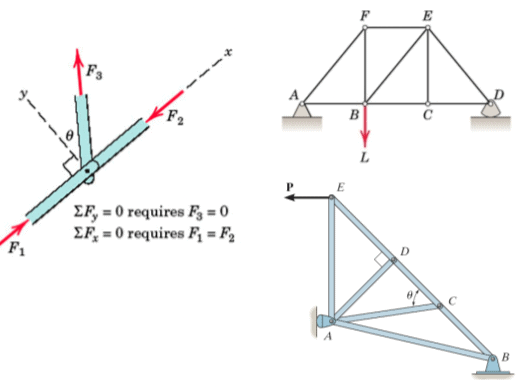Method of Section

• It can be used to determine three unknown member forces per FBD since all three equilibrium equations can be used
• Equilibrium under non-concurrent force system
• Not more than 3 members whose forces are unknown should be cut in a single section since we have only 3 independent equilibrium equations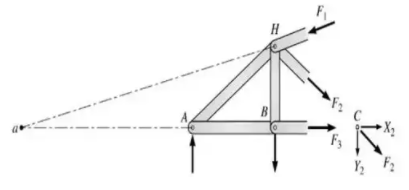Principle

• If a body is in equilibrium, then any part of the body is also in equilibrium.
• Forces in few particular member can be directly found out quickly without solving each joint of the truss sequentially
• Method of Sections and Method of Joints can be conveniently combined
• A section need not be straight.
• More than one section can be used to solve a given problem

Example 2: The truss in Fig given below is pinned to the wall at point F, and supported by a roller at point C. Calculate the force (tension or compression) in members BC, BE, and DE.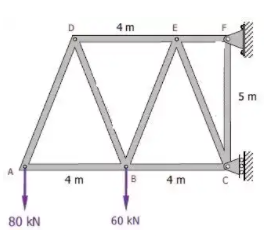Solution: From section to the left of a-a
[ΣFV = 0
5/√29 FBE = 80 + 60
FBE = 150.78 kN tension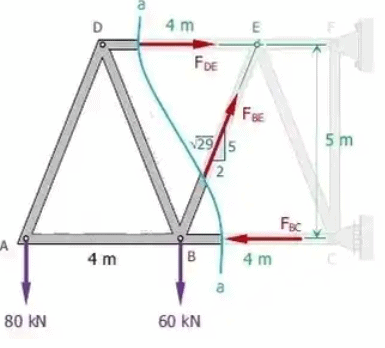ΣM= 0
5FBC = 6(80)+2(60)
FBC = 120 kN compression
ΣM= 0
5FDE = 4(80)
FDE = 64 kN Tension

Indeterminate Truss

(i) Final force in the truss member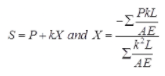sign convn → +ve for tension, –ve for compression
where,
S = Final force in the truss member
K = Force in the member when unit load is applied in the redundant member
L = Length of the member
A = Area of the member
E = Modulus of elasticity
P = Force in the member when truss become determinate after removing one of the member.
P = Zero for redundant member.
Lack of Fit in Truss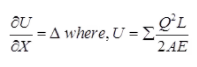Q = Force induce in the member due to that member which is 'Δ' too short or 'Δ' too long is pulled by force 'X'.

Deflection of Truss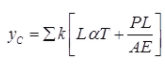Where, yC = Deflection of truss due to effect of loading & temp. both.
If effect of temperature is neglected then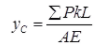α = Coefficient of thermal expansion
T = Change in temperature
T = +ve it temperature is increased
T = -ve it temperature is decreased
P & K have same meaning as mentioned above.

Offer running on EduRev: Apply code STAYHOME200 to get INR 200 off on our premium plan EduRev Infinity!

## Structural Analysis

30 videos|122 docs|28 tests

,

,

,

,

,

,

,

,

,

,

,

,

,

,

,

,

,

,

,

,

,

;